# AP Chemistry : Other Molecular Concepts

## Example Questions

### Example Question #1 : Molecules

Which of the following is an intrinsic physical property of a chemical substance?

Heat

Enthalpy

Viscosity

Mass

Viscosity

Explanation:

Intrinsic properties do not depend on the amount of a substance present. Mass, heat, and enthalpy are all dependent on the amount of substance.

### Example Question #1 : Molecules

Which of the following is NOT true of the law of constant composition?

It is what ensures that every sample of H2O will have 2 hydrogen atoms for every 1 oxygen atom.

It can be applied to solutions.

It states that a given compound will contain the same elements in an identical mass ratio.

It can be applied to pure samples.

It can be applied to solutions.

Explanation:

The law of constant composition can't be applied to solutions because it only applies to pure samples. Solutions are not pure samples, since they contain more than just 1 compound.

### Example Question #52 : Compounds And Molecules

A compound that is formed in the mechanism of a reaction but does not appear in the overall reaction is referred to as a(n) __________.

catalyst

product

intermediate

reactant

intermediate

Explanation:

This is the very definition of an intermediate. It is formed in one step within the mechanism of the reaction, but then something else happens to it as the reaction continues to completion; thus, it does not show up in the overall reaction.

### Example Question #4 : Molecules

A compound is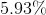hydrogen and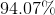oxygen by mass. What is the name and molecular formula of this compound?

Peroxide,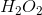Peroxide,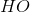None of these are correct

Alcohol,Alcohol,Peroxide,Explanation:

Knowing there is at least one hydrogen atom and one oxygen atom in this compound means the total molar mass must be at least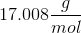. If we first assumeis equivalent to only 1 mole of hydrogen, we get a total molar mass of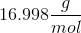. This number is too low to make logical sense.

Therefore, we must next assume there are 2 moles of hydrogen. Using this assumption, we can find the hypothetical molecular mass.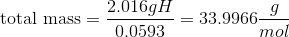Use this molecular mass to calculate the mass of oxygen.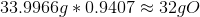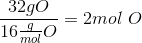Therefore, there must be 2 moles of hydrogen and 2 moles of oxygen, making the molecular formula.

This compound is called peroxide.

### Example Question #51 : Compounds And Molecules

A compound is comprised only of carbon and hydrogen. The molar mass of the compound is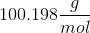and the compound is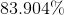carbon by mass. What is the empirical formula?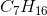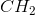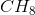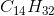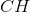Explanation:

Use the molar mass and percent by mass of carbon to find the moles of carbon in the compound.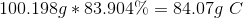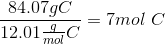We know that the compound is made solely of carbon and hydrogen. This allows us to find the percent by mass of hydrogen.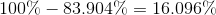Use the molar mass and the percent by mass of hydrogen to find moles of hydrogen in the compound.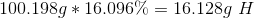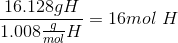The molecular formula is.

Because 7 and 16 have no common factors, the compound cannot be further reduced (in a numerical, not chemical sense).is also the empirical formula.

### Example Question #52 : Compounds And Molecules

A compound is comprised of sulfur and element X. The compound has a molar mass of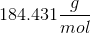and is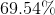sulfur. Analysis shows the compound has twice as much sulfur as element X. Find the molecular and empirical formulas for the compound, including the identity of element X.

Molecular: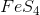Empirical: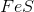Molecular:Empirical:Molecular: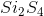Empirical: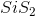Molecular: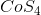Empirical:Molecular:Empirical:Molecular:Empirical:Explanation:

Use the molar mass and percent by mass of sulfur to find the moles of sulfur in the compound.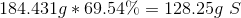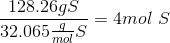Since the compound consists of only sulfur and element X, we can subtract to find the percent by mass of element X.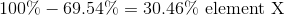Use the percent by mass to find the mass per mole of compound of element X.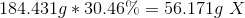Because we are told there are one-half as many moles of X as there are of sulfur, we know there are 2 moles of X (as compared to the 4 moles of sulfur). Divide the mass of element X by the moles of element X to find its atomic mass and its identity.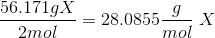By consulting a periodic table, we can find that element X is silicon.

Therefore, the molecular formula isand the empirical formula is.

### Example Question #53 : Compounds And Molecules

A compound is comprised of sodium and element X. The compound has a molar mass of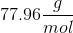and is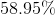sodium by mass. The compound consists of a 1:1 ratio between sodium and element X. Find the molecular and empirical formulas of the compound, including the identity of element X.

None of these are correct

Molecular: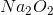Empirical: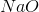Molecular: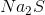Empirical:Molecular:Empirical:Molecular:Empirical: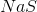Molecular:Empirical:Explanation:

Use the molar mass and percent by mass of sodium to find the moles of sodium in the compound.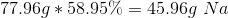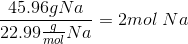Since the compound consists of only sodium and element X, we can subtract to find the percent by mass of element X.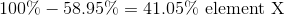Use the percent by mass to find the mass per mole of compound of element X.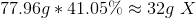Because there is a 1:1 ratio, between sodium and element X, they must have an equal number of moles in the compound. Since there are 2 moles of sodium, there must also be 2 moles of element X. Divide the mass of element X by the moles of element X to find its atomic mass and its identity.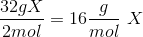By consulting a periodic table, we can find that element X is oxygen.

Therefore element X is Oxygen.

Therefore, the molecular formula isand the empirical formula is.